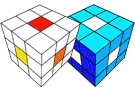﻿ Math 30 Worksheet 1 (Curriculum Alberta)

# Tutor Calgary

Lessons are exclusively online during the pandemic.
Reduced prices are applicable.### Math 30 Curriculum

(back to the general resource page for Math 30)

Textbook: Pre-Calculus 12

For extra practice use iWrite Math Pre-calculus 12 from AVP.

math 30-1 textbook alberta

math 30 solutions

math 30-1 textbook solutions

iwrite math pre calculus solutions pdf

Math 30-1 Explained